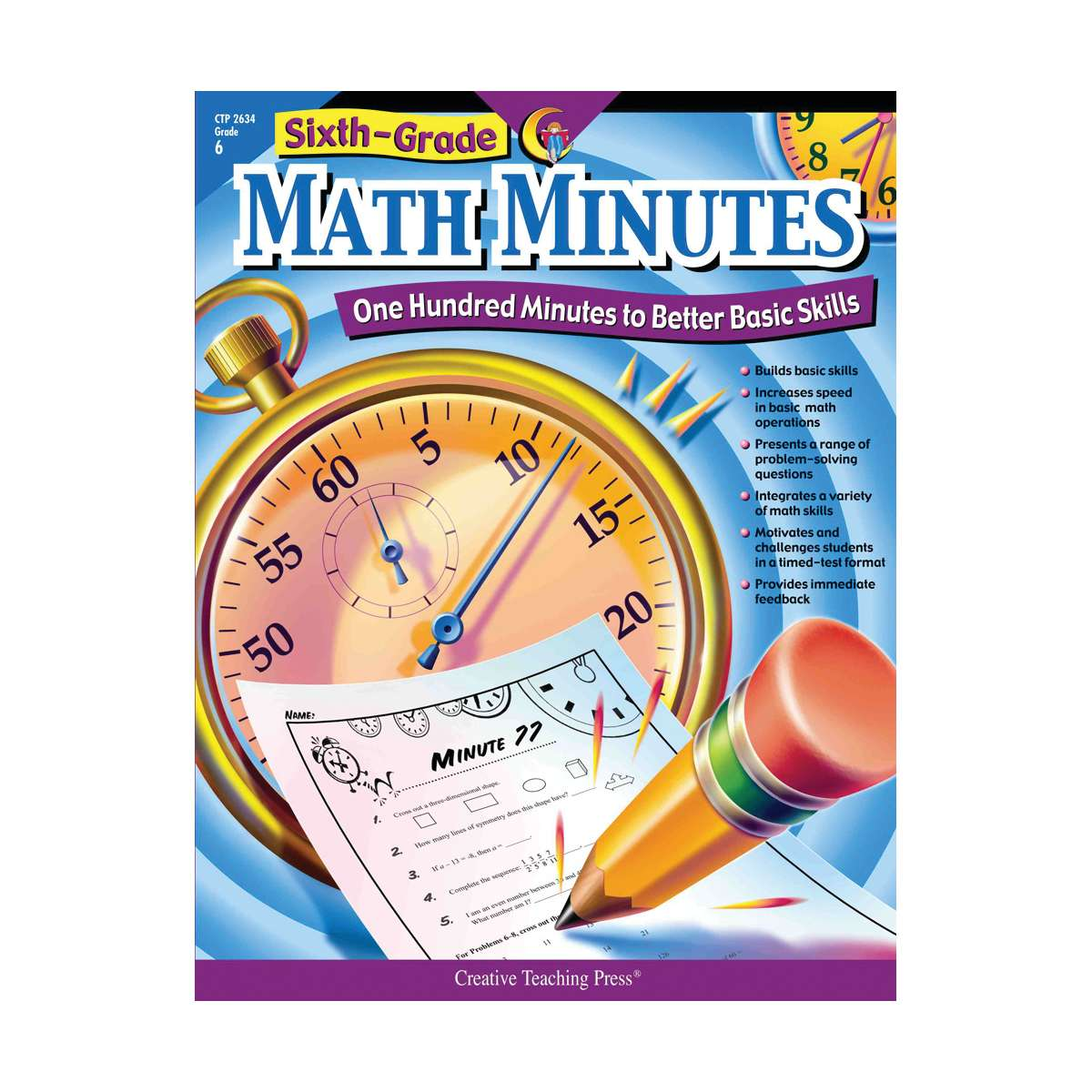•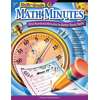•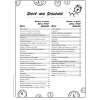•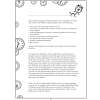•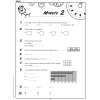•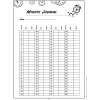•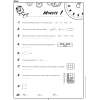•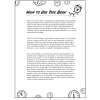•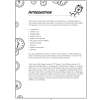Math Minutes, Grade 6

Product Number: CTP2634
\$16.99
Help middle-grade students master essential math skills with the motivating, classroom-tested Math Minutes format featured in...
Quantity

Help middle-grade students master essential math skills with the motivating, classroom-tested Math Minutes format featured in these two new books. Each book provides 100 “Minutes” of 10 problems each for students to complete within a one- to two-minute period. Providing daily practice in every key area of math instruction, Math Minutes is a fun way to improve students’ problem-solving speed (crucial for testing situations) and an instant assessment tool.

Now there's a way for students to practice their math skills in a timed situation, enhance overall math fluency, and have fun at the same time! Sixth-Grade Math Minutes provides students with practice in every key area of sixth-grade math instruction, including:

• multiplication
• division
• problem solving
• probability
• graphing
• place value
• algebra and functions
• geometry
• square roots
• percents, decimals, and fractions
• number sense
• measurement
• spatial connections
• math vocabulary
• and more!

Sixth-Grade Math Minutes features 100 "Minutes." Each Minute consists of 10 classroom-tested problems of varying degrees of difficulty for students to complete within a one-to two-minute period. This unique format offers students an ongoing opportunity to improve their own math fluency in a manageable, nonthreatening manner. The quick, timed drills, combined with instant feedback, make this a challenging and motivational assignment students will look forward to using each day. Students become active learners as they discover mathematical relationships, apply acquired understanding to complex situations, and find solutions to the realistic problems in each Minute.

CTP2634